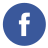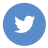### Java Arrays

java

In Java, an array is a container object that holds a fixed number of values of a single type. The length of an array is established when the array is created. After creation, its length is fixed.

Here is the syntax for creating an array in Java:

``````type[] arrayName; // creates an array reference
arrayName = new type[arraySize]; // creates an array of the specified size

// or, the two steps can be combined:
type[] arrayName = new type[arraySize];``````

Here is an example of creating and initializing an array of integers:

``````int[] numbers = new int; // creates an array of size 5

// initialize the elements of the array
numbers = 10;
numbers = 20;
numbers = 30;
numbers = 40;
numbers = 50;``````

You can also use the short form of array initialization:

``int[] numbers = {10, 20, 30, 40, 50};``

To access an element of an array, use the array index in square brackets:

``int x = numbers; // x is 30``

To iterate over the elements of an array, you can use a for loop:

``````for (int i = 0; i < numbers.length; i++) {
int n = numbers[i];
System.out.println(n);
}``````